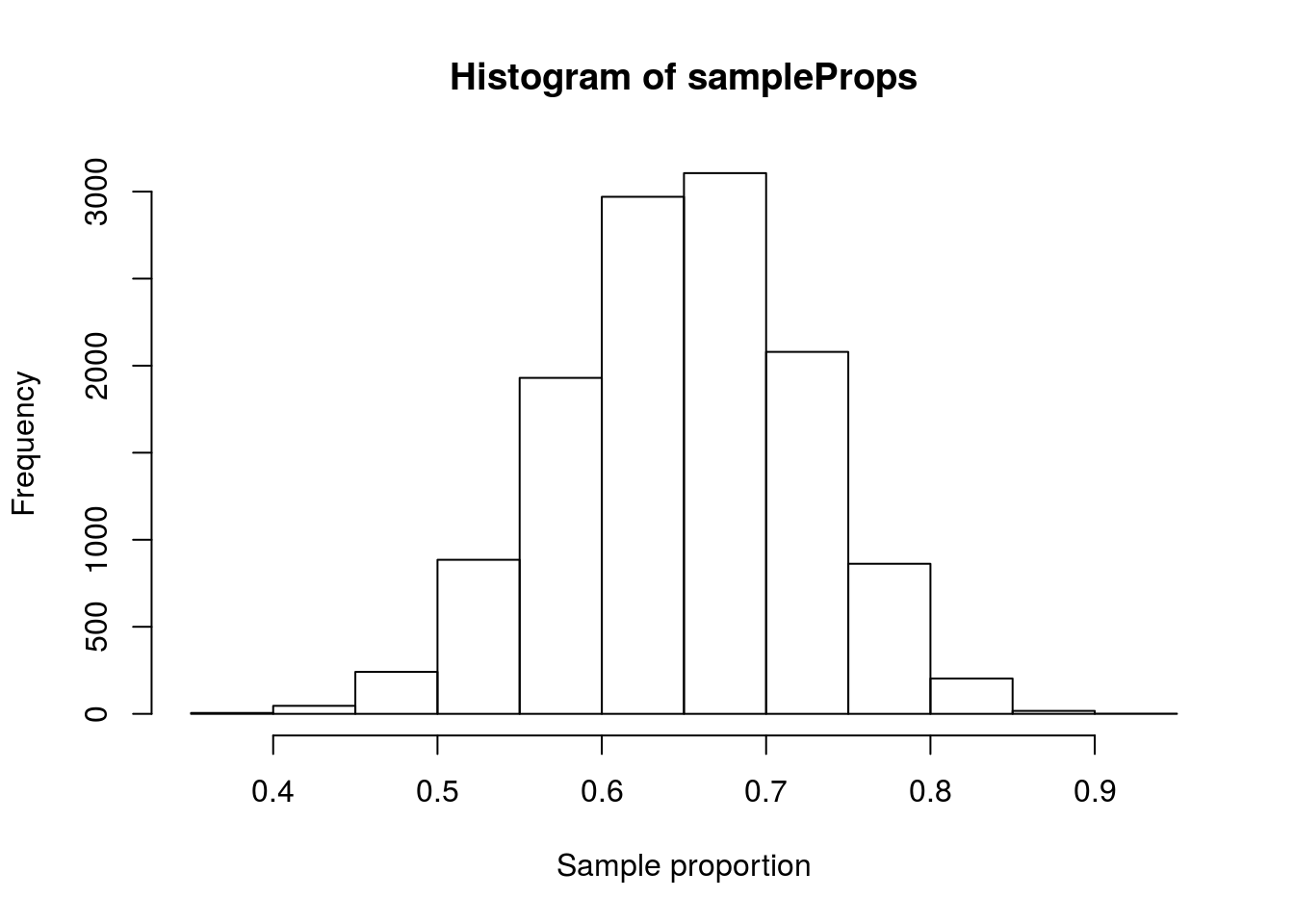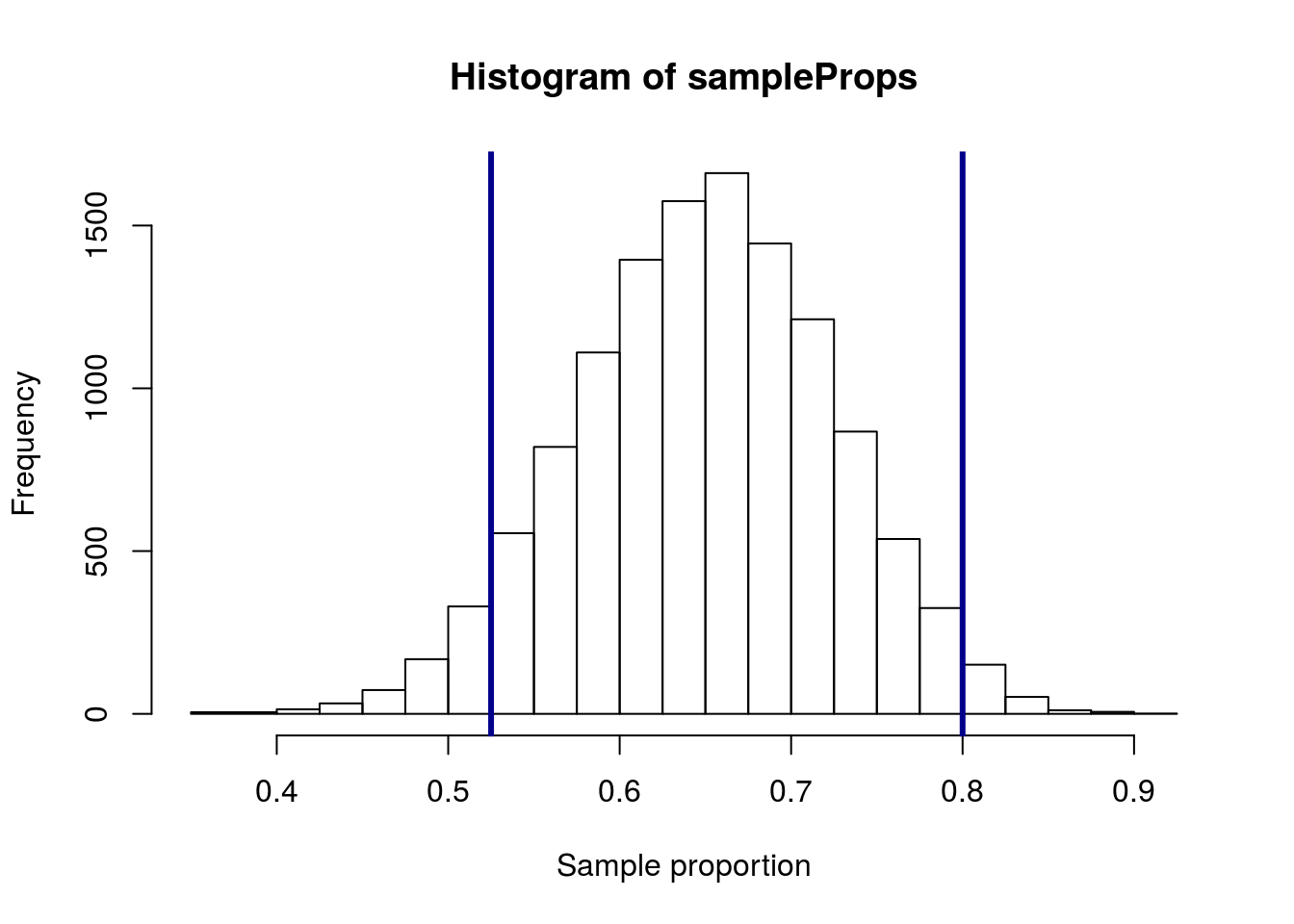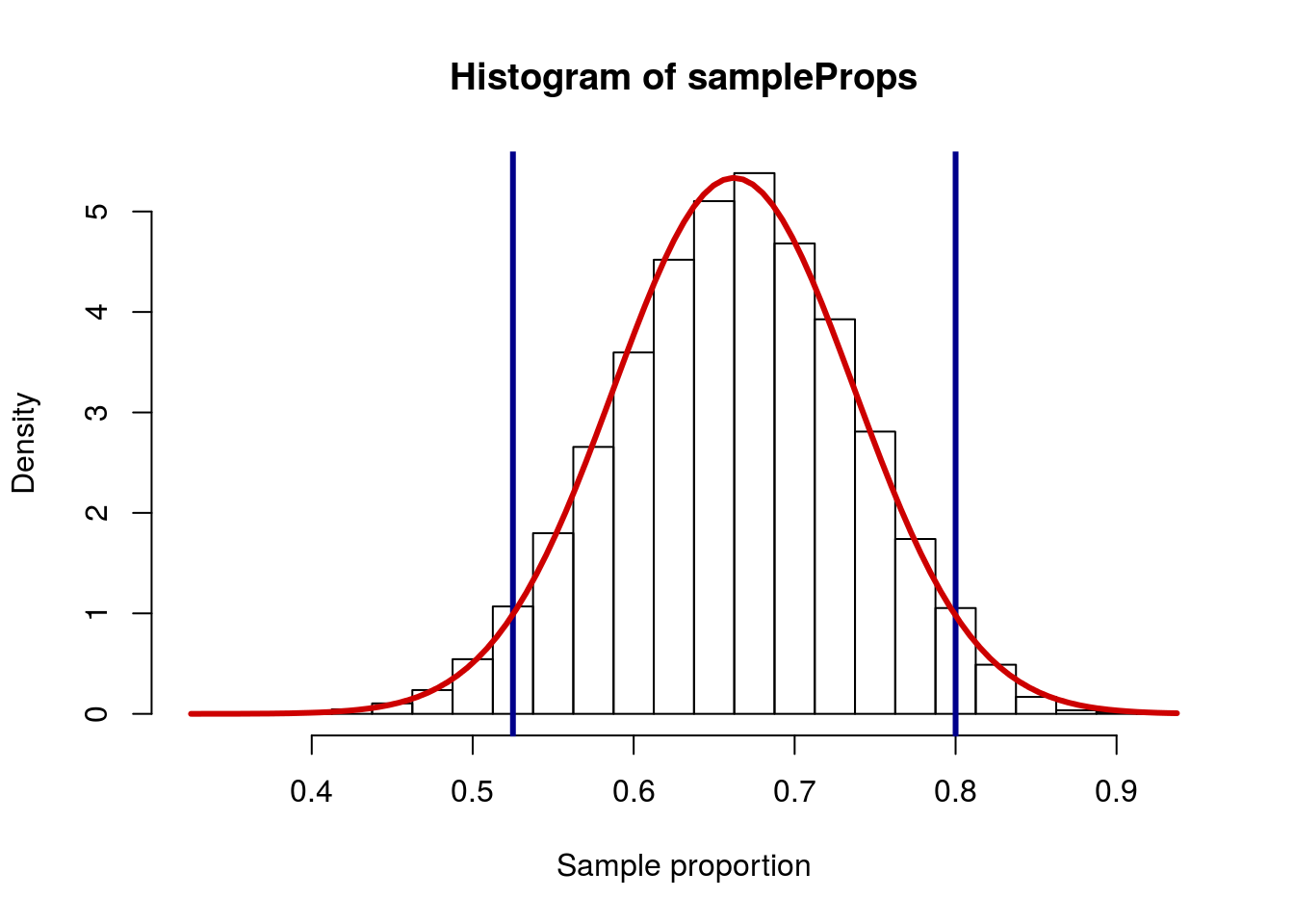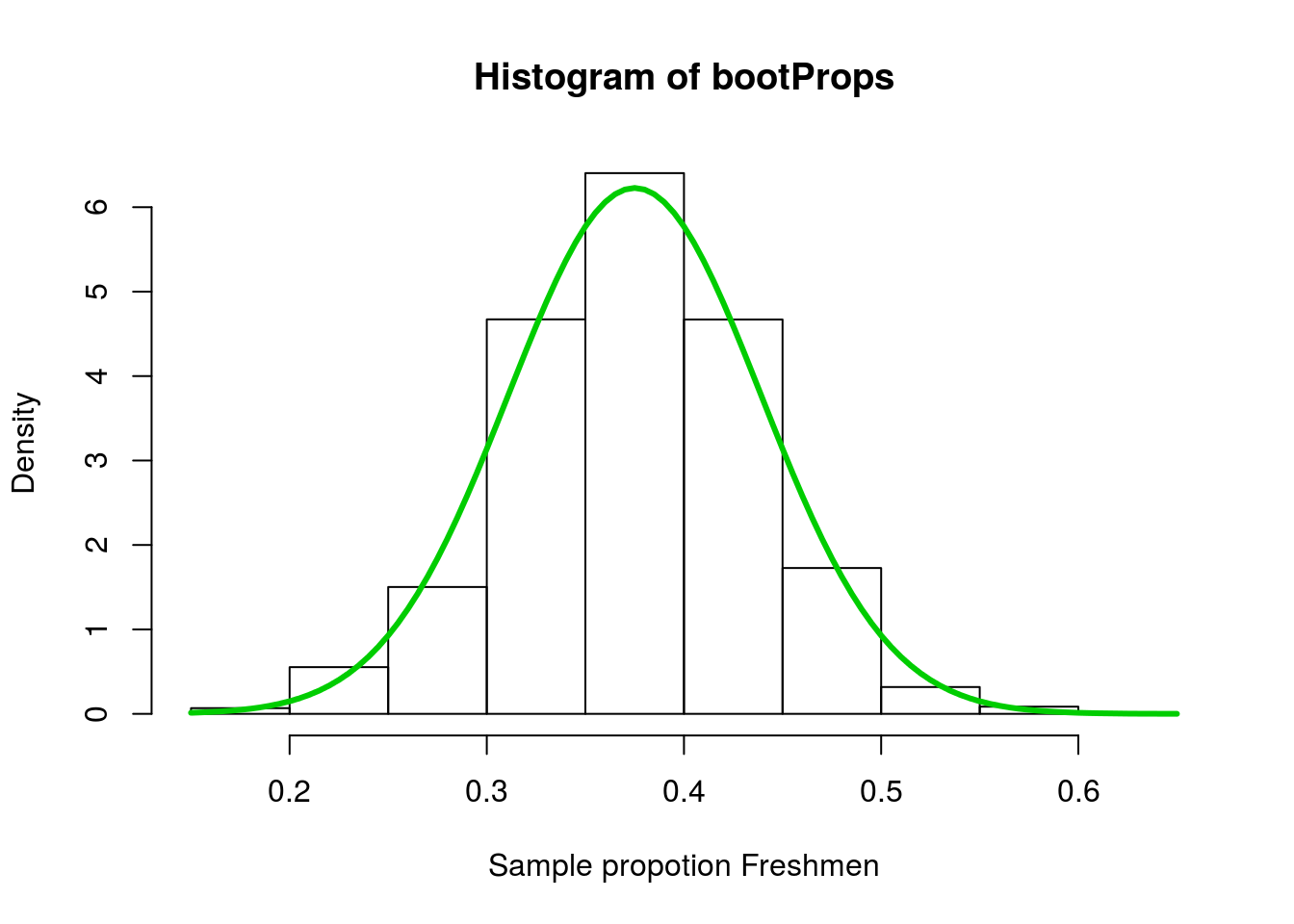# An introduction to statistics in R

### A series of tutorials by Mark Peterson for working in R

• NFLcombineData.csv
• Data on the Height, Weight, and position type of all 4299 players that participated in the NFL combine during the collection period
• survey.csv
• Data from intro stats students
• mlbCensus2014.csv
• Basic data on every Major League Baseball player on a 25-man roster as of 2014/May/15th
# Set working directory
# Note: copy the path from your old script to match your directories
setwd("~/path/to/class/scripts")

mlb <- read.csv("data/mlbCensus2014.csv")

## 20.2 Overview

Before we get back to estimating distributions with the normal approximation, let’s recall what it is that we are trying to simulate. For any one sample, we don’t know whether or not our particular sample is representative of the population. If we were able to, we could draw multiple samples from the population and see what they looked like.

## 20.3 Sampling distribution

So, let’s do that. Our nfl data is the full population of all players that atteneded the combine. We can create a lot of samples from it in order to see what they look like. Because we are talking about proportions, we’ll look at the proportion of participants that are skill players. First, let’s see what the true population parameter is.

# Calculate population parameter
popProp <- mean(nflCombine$positionType == "skill") popProp ##  0.662247 So, about two-thirds of the players are skill players. If we draw lots of samples, will we alwats see that proportion? We can test that by drawing many samples of 40 players, and measuring the proportion that are skill players. # Initialize variable sampleProps <- numeric() # Loop to draw many samples for(i in 1:12345){ # Save the proportion that are "skill" from a sample sampleProps[i] <- mean(sample(nflCombine$positionType,
40) == "skill")
}

Then, as always, we plot our result to make sure it make sense and to give us an idea of what we found.

# Visualize
hist(sampleProps
, xlab = "Sample proportion")As expected, we see a roughly normal distribution that is peaked around our sample proportion (0.662). However, notice that there are several samples that are quite different from that, as low as 0.35 and as high as 0.925. What we are, generally, interested in is where the middle chunk lies. For this, we calculate the quantiles, usually to catch the middle 95%.

# Where is the middle 95%?
quantile(sampleProps,
c(0.025, 0.975))
##  2.5% 97.5%
## 0.525 0.800

This tells us that, 95% of the time we sample 40 individuals from this population, our sample proportion will fall between 0.525 and 0.8. We can use this to help us make some inferences soon. If you’d like, you can add lines to your plot to mark those points (note that I also changed the breaks = just for the visual display).### 20.3.1 Using the normal model

Becuase the distribution is roughly normal, we can also apply the normal model to describe it. All we need to specify the normal is the mean, which we set to the population parameter when we know it, and the standard deviation, which we can calculate from the sample proportions.

# Calculate sd
sd(sampleProps)
##  0.0747751

Of note, this means that we expect 95% of samples to fall within about 0.15 of the true value (two times the sd). That gives us a range between 0.513 and 0.812, which is very similar to what we calculated with quantile() above. Now, let’s add the curve on top of the histogram.

curve(dnorm(x,
mean = popProp,
sd = sd(sampleProps)),
col = "red3",
lwd = 3)Of note: your line is likely to consitently hit the right edge of the bins on your distribution, rather than the center. That is because when R makes histograms, it uses the function pretty() to pick good looking break points. Unfortunately, those breaks are also at the same place where proportions out of round numbers (like 40), tend to land. Because R counts the values on the right edge of each bin, the position of the bin can look a little bit odd. I manually set (and adjusted) the breaks for the histogram above (not something I expect you to do) for illustrative purposes.

However, through all of this, we still had to contstruct the null distribution. So, what did we actually gain by applying the normal model?

## 20.4 Estimating Standard Deviation

What we gain from the application of this model is the opportunity to make a short cut. The sampling loops (sampling, bootstrap, and null) that we have been making are relatively simple to implement in R. However, that is only recently true. Through most of the history of statistics, such sampling would have been an immense undertaking. So, statisticians were forced to develop short cuts that would allow them to estimate the shape of sampling distributions.

What we need is to specify the normal distribution we want to use, which only requires a mean and a standard deviation. We have already seen that for a sampling distribution, we can (and should) just use the population proportion as our mean. But, how can we estimate the standard deviation?

It turns out that there is a relatively simple formula to estimate standard error of a proportion estimate which relies on nothing more than knowing the proportion calculated by our sample (p) and the number of samples we collected (n).

$SE = \sqrt{\frac{p \times (1-p)}{n}}$

In R, this looks like:

SE <- sqrt( ( p*(1-p) ) / n )

In words, that is the square root of p times 1-p divided by n.

At least some of this should make sense to us. We have already seen that as sample size increases, SE decreases, exactly what the denominator shows. The rest of the derivation is beyond the scope of this course: for now, you will just need to trust that it is valid.

### 20.4.1 Applying the shortcut

We can compare this shortcut to the NFL sampling data to see how accurate it is.

# Calculate the sd by formula
formSD <- sqrt( ( popProp*(1-popProp) ) / 40 )
formSD
##  0.07477899

Which is incredibly close to the value we calculated from the sampling distribition.

# Compare to sd of samples
sd(sampleProps)
##  0.0747751

And we can see that they are incredibly similar. This means that we can use this calculated standard error to estimate any interval we want. Here, we can compare the 90% interval using the two methods.

# Calculate 90% interval
qnorm(c(0.05,0.95),
mean = popProp,
sd   = formSD)
##  0.5392465 0.7852475
# Compare
quantile(sampleProps,
c(0.05,0.95))
##    5%   95%
## 0.525 0.775

As above, this tells us that 90% of samples (of size 40) taken from the NFL Combine data will give sample proportions inside these intervals. The slight difference is largely due to the discrete nature of the sample data. In reality, the sample proportions can only take on 41 values ($$\frac{0}{40}$$ to $$\frac{40}{40}$$), so there is a limite to where the quantiles can give us cutoffs.

Treating the THW column from the mlb data, create a sampling distribution of the proportion of players that throw left-handed (mlb$THW == "L"). Use a sample size of 50 players. Then, calculate the standard error using the formula above and compare it to the standard deviation of the sampled values. You should get a standard error of 0.0573. ## 20.5 Calculating confidence interval To calculate a confidence interval (CI) from a sample, we had been using bootstrapping. When we bootstrap, we are using our original sample as representative of the original population. As long as the sample is sort of the same as the original population, the width of our bootstrap distribution will be very similar to the width of the true sampling distribution. This tells us how far we’d have to “reach” to be confident that we are catching the true mean. Since we don’t know which direction we are wrong in (if we are at all), we say that we are confident that our true population mean falls somewhere in that range. To see this in action, let’s ask what proportion of students that responded to the survey are Freshmen. # Look at the data table(survey$class)
##
##  Freshman    Junior    Senior Sophomore
##        21        10         6        19

So, we see that 21 of the 56 are Freshmen. Since we are interested in the proportion, that is what we will calculate. Recall that mean() here is just counting up all of the Freshmen, then dividing by the number of students.

# Calculate the sample proportion
mySampleProp <- mean(survey$class == "Freshman") mySampleProp ##  0.375 Next, we need to construct a bootstrap distribution and look at its spread. # initialize new variable bootProps <- numeric() # Loop to draw many samples for(i in 1:15486){ bootProps[i] <- mean(sample(survey$class,
replace = TRUE) == "Freshman")
}

You will notice that this loop looks a lot like the loop above. It is just copied with slight changes to work from these data instead. Then, visualize it. I am including the curve directly this time.

# Visualize
hist(bootProps,
xlab = "Sample propotion Freshmen",
freq = FALSE)### 20.5.1 Apply the standard deviation shortcut

Next, we want to estimate the standard error of the distribution. Let’s jump straight to the formula this time:

# Estimate standard error
classSD <- sqrt( ( mySampleProp*(1-mySampleProp)
) / nrow(survey) )
classSD
##  0.06469365

How does this compare to the standard error from our sample values?

# Calculate sd from samples
sd(bootProps)
##  0.06405583

Quite close. So, we can use this estimated standard error to add the curve to the plot.

# Add the normal curve
curve(dnorm(x,
mean = mySampleProp,
sd   = sd(bootProps)),
col = "green3", lwd = 3,
add = TRUE)Finally, what we started off trying to do: let’s calculate the 90% confidence interval using each method. We use quantile() to assess the distribution and qnorm() to work from the normal model.

# show the CI cutoffs using the distribution
quantile(bootProps,
c(0.05, 0.95))
##        5%       95%
## 0.2678571 0.4821429
# Compare to CI from normal model
qnorm(c(0.05, 0.95),
mean = mySampleProp,
sd   = classSD)
##  0.2685884 0.4814116

This tells us that, because we are assuming the population is roughly similar to the sample, we expect that the same shape would have been created by the population, so 90% of the time our sample should not be more wrong than the width of the CI. In simpler terms: it tells us that our true population proportion is likely to fall within the CI 90% of the time.

Specifically for this class, we are 90% confident that the true population proportion of Freshman in intro stats courses at Viterbo is between 0.269 and 0.481.

### 20.5.2 Try it out

Based on the sugaryDrink column of the survey data, calculate the 99% confidence interval for the proportion of intro statistics students at Viterbo that call a “sugary carbonated beverage” a “Pop”.

You do not need to use a bootstrap sample, though you can do one if you want to confirm your answer. You should get a confidence interval of 0.241 to 0.58

## 20.6 Using the standard normal model

In all of the above examples, we have used the the mean = and sd = arguments to qnorm() to specify which normal model we were talking about. However, historically, that was not possible. If you wanted to know a quantile cut off, you looked it up in a massive table (See this Wikipedia example). The table only had enteries at a selected points, and so was less specific.

Further, because there are an infinite number of possible normal distributions (any mean and any standard deviation), only one table was printed: the standard normal. This meant that to use it, it was neccessary to convert values to values back and forth from the normal model.

This has some advantages, and is also necessary for working with some of the distributions we will encounter soon. The biggest advantage is that it shows that the cut-offs for a given CI are always very closely related.

The value we find in this way is called the z-critical value, as it is the cutoff point on the z-scale (the abbreviation for the standard normal). It is generally written as z*.

So, if we want to caluculate a 90% CI, we can find the z* values using the standard normal:

# Find z* values
zCrit <- qnorm( c(0.05,0.95))
zCrit
##  -1.644854  1.644854

This tells us how many standard deviations above and below the mean we need to include to capture 90% of the distribution. To use this on our bootstrap example from above, we simply add and subtract 1.645 standard deviations from the mean.

# Calculate CI from z*
mySampleProp + zCrit*formSD
##  0.2519995 0.4980005

Which we can see is exactly the same as if we calculate it directly. 

qnorm(c(0.05,0.95),
mean = mySampleProp,
sd   = formSD)
##  0.2519995 0.4980005

Mathematically we can see why this works because the z score of a value can be calculated as:

$z_i = \frac{x_i - \bar{x}}{\sigma_x}$

Where x is a numeric variable, i represents the index (so $$x_i$$ is the ith value of x), $$\bar{x}$$ is the mean of x, and $$\sigma_x$$ is the standard deviation of x.

This means that we can solve for xi to get:

$x_i = \bar{x} + (z_i \times \sigma_x)$

Finally, we can check on our 95% rule this way. What are the z* values for a 95% CI?

# Find z* values for 95% CI
qnorm( c(0.025,0.975))
##  -1.959964  1.959964

These values are close, but not exactly the value of 2 we were using. So, 95% CI’s calculated directly will be more accurate than our rule of thumb of 2 times the standard error. However, that rule is still a useful first approximation.

It may seem that this is an odd thing to have to do when we can already calculate CI’s directly. However, the z* values play a crucial role in some calculations that we will see soon, and a closely related form is required when we start working with means. In addition, the historical use of these approaches ensures that you will encounter some mention of critical values in other statistics (either resources or publications you read).

### 20.6.1 Try it out

Calculate the z-critical (z*) values for a 99% CI. Use them to calculate a 99% CI of the intro statistics students that use the word “Pop”. Compare it to your previous try it our to confirm that it worked.